# Types of Binary Tree

A tree is said to be binary tree when,

1. A binary tree has a root node. It may not have any child nodes(0 child nodes, NULL tree).

2. A root node may have one or two child nodes. Each node forms a binary tree itself.

3. The number of child nodes cannot be more than two.

4. It has a unique path from the root to every other node.

There are four types of binary tree:

1. Full Binary Tree
2. Complete Binary Tree
3. Skewed Binary Tree
4. Extended Binary Tree

#### 1. Full Binary Tree

• If each node of binary tree has either two children or no child at all, is said to be a Full Binary Tree.
• Full binary tree is also called as Strictly Binary Tree.

•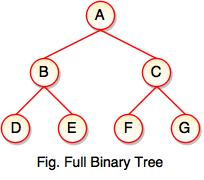• Every node in the tree has either 0 or 2 children.
• Full binary tree is used to represent mathematical expressions.

#### 2. Complete Binary Tree

• If all levels of tree are completely filled except the last level and the last level has all keys as left as possible, is said to be a Complete Binary Tree.
• Complete binary tree is also called as Perfect Binary Tree.

•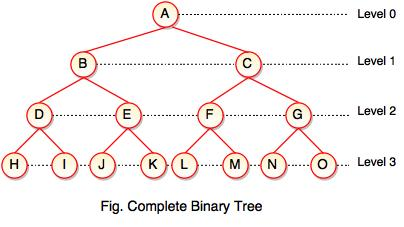• In a complete binary tree, every internal node has exactly two children and all leaf nodes are at same level.
• For example, at Level 2, there must be 22 = 4 nodes and at Level 3 there must be 23 = 8 nodes.

#### 3. Skewed Binary Tree

• If a tree which is dominated by left child node or right child node, is said to be a Skewed Binary Tree.
• In a skewed binary tree, all nodes except one have only one child node. The remaining node has no child.

•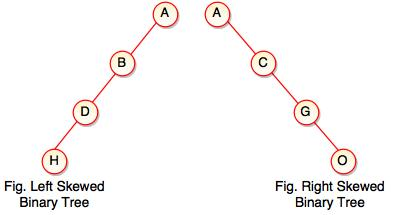• In a left skewed tree, most of the nodes have the left child without corresponding right child.
• In a right skewed  tree, most of the nodes have the right child without corresponding left child.

#### 4. Extended Binary Tree

• Extended binary tree consists of replacing every null subtree of the original tree with special nodes.
• Empty circle represents internal node and filled circle represents external node.
• The nodes from the original tree are internal nodes and the special nodes are external nodes.
• Every internal node in the extended binary tree has exactly two children and every external node is a leaf. It displays the result which is a complete binary tree.## AVL Tree

• AVL tree is a height balanced tree.
• It is a self-balancing binary search tree.
• AVL tree is another balanced binary search tree.
• It was invented by Adelson-Velskii and Landis.
• AVL trees have a faster retrieval.
• It takes O(logn) time for addition and deletion operation.
• In AVL tree, heights of left and right subtree cannot be more than one for all nodes.

•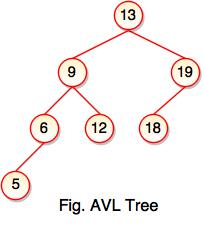• The above tree is AVL tree because the difference between heights of left and right subtrees for every node is less than or equal to 1.

•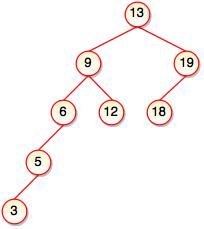• The above tree is not AVL because the difference between heights of left and right subtrees for 9 and 19 is greater than 1.
• It checks the height of the left and right subtree and assures that the difference is not more than 1. The difference is called balance factor.